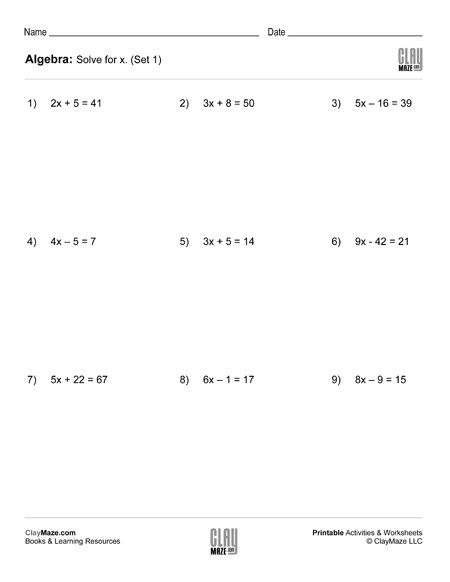# Solve the Equations I (Set 1)

This is a worksheet for students learning how to solve equations. Each problem has a combination of multiplication and either addition or subtraction, but division will also need to be used to find the final answer.